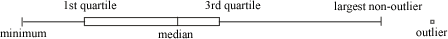index: click on a letter A B C D E F G H I J K L M N O P Q R S T U V W X Y Z A to Z index index: subject areas numbers & symbols sets, logic, proofs geometry algebra trigonometry advanced algebra & pre-calculus calculus advanced topics probability & statistics real world applications multimedia entrieswww.mathwords.com about mathwords website feedback

 Boxplot Modified Boxplot A data display that shows the five-number summary. The whiskers, stretching outward from the first quartile and third quartile as shown below, are no longer than 1.5 times the interquartile range (IQR). Outliers beyond that are marked separately. Note: Beginners are sometimes taught to draw box-and-whisker plots, which do not show outliers. Modified boxplot is a name sometimes used for boxplots to distinguish them from box-and-whisker plots.See also